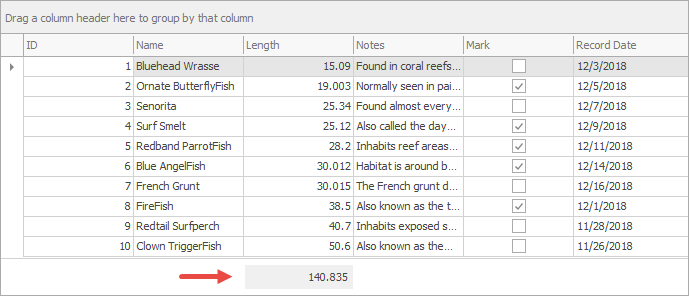# How To: Calculate Custom Summaries Based on Column Summary Items

The example below creates a new custom summary items and populates it on the GridView.CustomSummaryCalculate event. You can test this sample in our DevExpress Demo Center (the link requires Demo Center of version 16.2 or newer to be installed).The custom summary item is located under the “Length” column. To add it, set up the GridColumn.SummaryItem property.

``````gridView.OptionsView.ShowFooter = true;
GridColumn column = gridView.Columns["Length"];
column.SummaryItem.SummaryType = SummaryItemType.Custom;
``````

The CustomSummaryCalculate event handler checks the current calculation state by reading the CustomSummaryEventArgs.SummaryProcess value. Cell values are added to the total sum only if the corresponding cell under the “Mark” column is checked.

``````double sum = 0;
gridView.CustomSummaryCalculate += (sender, e) => {
GridView view = sender as GridView;
if (e.IsTotalSummary && (e.Item as GridSummaryItem).FieldName=="Length") {
GridSummaryItem item = e.Item as GridSummaryItem;
if (item.FieldName == "Length") {
switch (e.SummaryProcess) {
case CustomSummaryProcess.Start:
sum = 0;
break;
case CustomSummaryProcess.Calculate:
bool shouldSum = (bool)view.GetRowCellValue(e.RowHandle, "Mark");
if (shouldSum) {
sum += (double)e.FieldValue;
}
break;
case CustomSummaryProcess.Finalize:
e.TotalValue = sum;
break;
}
}
}
};
``````

To automatically update the summary item when users check or clear the “Mark” column checkboxes, the GridView.UpdateTotalSummary method is called on the RepositoryItem.EditValueChanged event.

``````RepositoryItemCheckEdit edit = gridView.Columns["Mark"].RealColumnEdit as RepositoryItemCheckEdit;
edit.EditValueChanged += (sender, e) => {
//Post an editor's value to a data source
gridView.PostEditor();
//Force calculation of the total summary
gridView.UpdateTotalSummary();
};
``````

The code below is a complete example code.

``````    // Handle this event to calculate summary values manually
double sum = 0;
gridView.CustomSummaryCalculate += (sender, e) => {
GridView view = sender as GridView;
if (e.IsTotalSummary && (e.Item as GridSummaryItem).FieldName=="Length") {
GridSummaryItem item = e.Item as GridSummaryItem;
if (item.FieldName == "Length") {
switch (e.SummaryProcess) {
case CustomSummaryProcess.Start:
sum = 0;
break;
case CustomSummaryProcess.Calculate:
bool shouldSum = (bool)view.GetRowCellValue(e.RowHandle, "Mark");
if (shouldSum) {
sum += (double)e.FieldValue;
}
break;
case CustomSummaryProcess.Finalize:
e.TotalValue = sum;
break;
}
}
}
};

gridView.OptionsView.ShowFooter = true;
GridColumn column = gridView.Columns["Length"];
column.SummaryItem.SummaryType = SummaryItemType.Custom;

RepositoryItemCheckEdit edit = gridView.Columns["Mark"].RealColumnEdit as RepositoryItemCheckEdit;
edit.EditValueChanged += (sender, e) => {
//Post an editor's value to a data source
gridView.PostEditor();
//Force calculation of the total summary
gridView.UpdateTotalSummary();
};
``````